# Selina solutions for Class 6 Mathematics chapter 28 - Polygons [Latest edition]

#### Chapters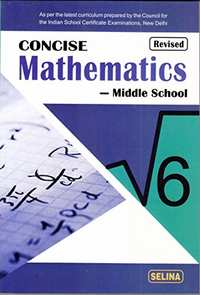## Chapter 28: Polygons

Exercise 28 (A)Exercise 28 (B)
Exercise 28 (A)

### Selina solutions for Class 6 Mathematics Chapter 28 Polygons Exercise 28 (A)

Exercise 28 (A) | Q 1.1

State, whether the following figure is a polygon or not: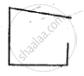• Yes

• No

Exercise 28 (A) | Q 1.2

State, whether the following figure is a polygon or not: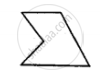• Yes

• No

Exercise 28 (A) | Q 1.3

State, whether the following figure is a polygon or not: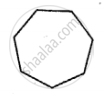• Yes

• No

Exercise 28 (A) | Q 1.4

State, whether the following figure is a polygon or not: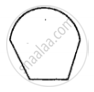• Yes

• No

Exercise 28 (A) | Q 1.5

State, whether the following figure is a polygon or not: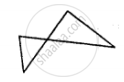• Yes

• No

Exercise 28 (A) | Q 2.1

Find the sum of interior angle of a polygon with: 9 sides

Exercise 28 (A) | Q 2.2

Find the sum of interior angle of a polygon with: 13 sides

Exercise 28 (A) | Q 2.3

Find the sum of interior angle of a polygon with: 16 sides

Exercise 28 (A) | Q 3.1

Find the number of side of a polygon, if the sum of its interior angle is: 1440°

Exercise 28 (A) | Q 3.2

Find the number of sides in a polygon if the sum of its interior angle is: 1620°

Exercise 28 (A) | Q 4

Is it possible to have a polygon, whose sum of interior angles is 1030°.

Exercise 28 (A) | Q 5.1

If all the angles of a hexagon are equal, find the measure of each angle.

Exercise 28 (A) | Q 5.2

If all the angles of an octagon are equal, find the measure of each angle.

Exercise 28 (A) | Q 6

One angle of a quadrilateral is 90° and all other angles are equal ; find each equal angle.

Exercise 28 (A) | Q 7

If angles of quadrilateral are in the ratio 4 : 5 : 3 : 6 ; find each angle of the quadrilateral.

Exercise 28 (A) | Q 8

If one angle of a pentagon is 120° and each of the remaining four angles is x°, find the magnitude of x.

Exercise 28 (A) | Q 9

The angles of a pentagon are in the ratio 5 : 4 : 5 : 7 : 6 ; find each angle of the pentagon.

Exercise 28 (A) | Q 10

Two angles of a hexagon are 90° and 110°. If the remaining four angles arc equal, find each equal angle.

Exercise 28 (B)

### Selina solutions for Class 6 Mathematics Chapter 28 Polygons Exercise 28 (B)

Exercise 28 (B) | Q 1

Fill in the blanks:

In case of regular polygon, with

 Number of sides Each exterior angle Each interior angle (i) 6 _________ __________ (ii) 8 _________ __________ (iii)  ________ 36° __________ (iv)  _______ 20° __________ (v)  _________ _________ 135° (vi) ________ _________ 165°
Exercise 28 (B) | Q 2.1

Find the number of sides in a regular polygon, if its interior angle is: 160°

Exercise 28 (B) | Q 2.2

Find the number of sides in a regular polygon, if its interior angle is: 150°

Exercise 28 (B) | Q 3.1

Find a number of side in a regular polygon, if it exterior angle is: 30°.

Exercise 28 (B) | Q 3.2

Find number of side in a regular polygon, if it exterior angle is: 36

Exercise 28 (B) | Q 4.1

Is it possible to have a regular polygon whose interior angle is: 135°

Exercise 28 (B) | Q 4.2

Is it possible to have a regular polygon whose interior angle is: 155°

Exercise 28 (B) | Q 5.1

Is it possible to have a regular polygon whose exterior angle is: 100°

Exercise 28 (B) | Q 5.2

Is it possible to have a regular polygon whose exterior angle is: 36°

Exercise 28 (B) | Q 6

The ratio between the interior angle and the exterior angle of a regular polygon is 2: 1. Find:

(i) each exterior angle of the polygon ;
(ii) number of sides in the polygon.

## Chapter 28: Polygons

Exercise 28 (A)Exercise 28 (B)## Selina solutions for Class 6 Mathematics chapter 28 - Polygons

Selina solutions for Class 6 Mathematics chapter 28 (Polygons) include all questions with solution and detail explanation. This will clear students doubts about any question and improve application skills while preparing for board exams. The detailed, step-by-step solutions will help you understand the concepts better and clear your confusions, if any. Shaalaa.com has the CISCE Class 6 Mathematics solutions in a manner that help students grasp basic concepts better and faster.

Further, we at Shaalaa.com provide such solutions so that students can prepare for written exams. Selina textbook solutions can be a core help for self-study and acts as a perfect self-help guidance for students.

Concepts covered in Class 6 Mathematics chapter 28 Polygons are Concept of Polygons - Side, Vertex, Adjacent Sides, Adjacent Vertices and Diagonal, Simple Polygons (Upto Octagons Regulars as Well as Non-regular)., Sum of Angles of a Polynomial, Sum of Exterior Angles of a Polynomial, Regular Polynomial.

Using Selina Class 6 solutions Polygons exercise by students are an easy way to prepare for the exams, as they involve solutions arranged chapter-wise also page wise. The questions involved in Selina Solutions are important questions that can be asked in the final exam. Maximum students of CISCE Class 6 prefer Selina Textbook Solutions to score more in exam.

Get the free view of chapter 28 Polygons Class 6 extra questions for Class 6 Mathematics and can use Shaalaa.com to keep it handy for your exam preparation